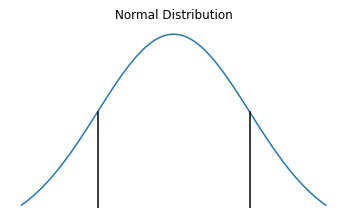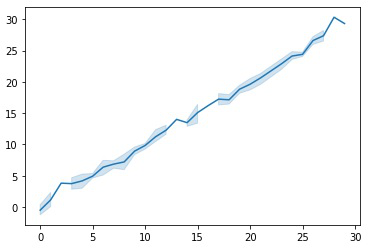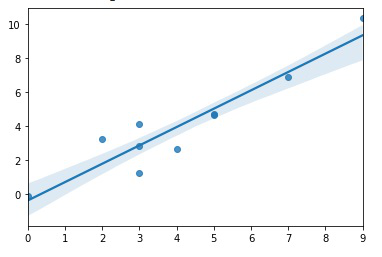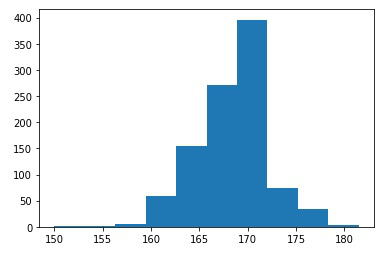# How to Plot a Confidence Interval in Python?

• Last Updated : 03 Jan, 2021

Confidence Interval is a type of estimate computed from the statistics of the observed data which gives a range of values that’s likely to contain a population parameter with a particular level of confidence.

A confidence interval for the mean is a range of values between which the population mean possibly lies. If I’d make a weather prediction for tomorrow of somewhere between -100 degrees and +100 degrees, I can be 100% sure that this will be correct. However, if I make the prediction to be between 20.4 and 20.5 degrees Celsius, I’m less confident. Note how the confidence decreases, as the interval decreases. The same applies to statistical confidence intervals, but they also rely on other factors.

Attention geek! Strengthen your foundations with the Python Programming Foundation Course and learn the basics.

To begin with, your interview preparations Enhance your Data Structures concepts with the Python DS Course. And to begin with your Machine Learning Journey, join the Machine Learning - Basic Level Course

A 95% confidence interval, will tell me that if we take an infinite number of samples from my population, calculate the interval each time, then in 95% of those intervals, the interval will contain the true population mean. So, with one sample we can calculate the sample mean, and from there get an interval around it, that most likely will contain the true population mean.Area under the two black lines shows the 95% confidence interval

Confidence Interval as a concept was put forth by Jerzy Neyman in a paper published in 1937. There are various types of the confidence interval, some of the most commonly used ones are: CI for mean, CI for the median, CI for the difference between means, CI for a proportion and CI for the difference in proportions.

Let’s have a look at how this goes with Python.

## Computing C.I given the underlying distribution using lineplot()

The lineplot() function which is available in Seaborn, a data visualization library for Python is best to show trends over a period of time however it also helps in plotting the confidence interval.

Syntax:

sns.lineplot(x=None, y=None, hue=None, size=None, style=None, data=None, palette=None, hue_order=None, hue_norm=None, sizes=None, size_order=None, size_norm=None, dashes=True, markers=None, style_order=None, units=None, estimator=’mean’, ci=95, n_boot=1000, sort=True, err_style=’band’, err_kws=None, legend=’brief’, ax=None, **kwargs,)

Parameters:

• x, y: Input data variables; must be numeric. Can pass data directly or reference columns in data.
• hue: Grouping variable that will produce lines with different colors. Can be either categorical or numeric, although color mapping will behave differently in latter case.
• style: Grouping variable that will produce lines with different dashes and/or markers. Can have a numeric dtype but will always be treated as categorical.
• data: Tidy (“long-form”) dataframe where each column is a variable and each row is an observation.
• markers: Object determining how to draw the markers for different levels of the style variable.
• legend: How to draw the legend. If “brief”, numeric “hue“ and “size“ variables will be represented with a sample of evenly spaced values.

Return: The Axes object containing the plot.

By default, the plot aggregates over multiple y values at each value of x and shows an estimate of the central tendency and a confidence interval for that estimate.

Example:

## Python3

 `# import libraries``import` `numpy as np``import` `seaborn as sns``import` `matplotlib.pyplot as plt`` ` `# generate random data``np.random.seed(``0``)``x ``=` `np.random.randint(``0``, ``30``, ``100``)``y ``=` `x``+``np.random.normal(``0``, ``1``, ``100``)`` ` `# create lineplot``ax ``=` `sns.lineplot(x, y)`

In the above code, variable x will store 100 random integers from 0 (inclusive) to 30 (exclusive) and variable y will store 100 samples from the Gaussian (Normal) distribution which is centred at 0 with spread/standard deviation 1. NumPy operations are usually done on pairs of arrays on an element-by-element basis. In the simplest case, the two arrays must have exactly the same shape, as in the above example. Finally, a lineplot is created with the help of seaborn library with 95% confidence interval by default. The confidence interval can easily be changed by changing the value of the parameter ‘ci’ which lies within the range of [0, 100], here I have not passed this parameter hence it considers the default value 95.The light blue shade indicates the confidence level around that point if it has higher confidence the shaded line will be thicker.

## Computing C.I. given the underlying distribution using regplot()

The seaborn.regplot() helps to plot data and a linear regression model fit. This function also allows plotting the confidence interval.

Syntax:

seaborn.regplot( x,  y,  data=None, x_estimator=None, x_bins=None,  x_ci=’ci’, scatter=True, fit_reg=True, ci=95, n_boot=1000, units=None, order=1, logistic=False, lowess=False, robust=False, logx=False, x_partial=None, y_partial=None, truncate=False, dropna=True, x_jitter=None, y_jitter=None, label=None, color=None, marker=’o’,    scatter_kws=None, line_kws=None, ax=None)

Parameters: The description of some main parameters are given below:

• x, y: These are Input variables. If strings, these should correspond with column names in “data”. When pandas objects are used, axes will be labeled with the series name.
• data:  This is dataframe where each column is a variable and each row is an observation.
• lowess: (optional) This parameter take boolean value. If “True”, use “statsmodels” to estimate a nonparametric lowess model (locally weighted linear regression).
• color: (optional) Color to apply to all plot elements.
• marker: (optional) Marker to use for the scatterplot glyphs.

Return: The Axes object containing the plot.

Basically, it includes a regression line in the scatterplot and helps in seeing any linear relationship between two variables. Below example will show how it can be used to plot confidence interval as well.

Example:

## Python3

 `# import libraries``import` `numpy as np``import` `seaborn as sns``import` `matplotlib.pyplot as plt`` ` `# create random data``np.random.seed(``0``)``x ``=` `np.random.randint(``0``, ``10``, ``10``)``y ``=` `x``+``np.random.normal(``0``, ``1``, ``10``)`` ` `# create regression plot``ax ``=` `sns.regplot(x, y, ci``=``80``)`

The regplot() function works in the same manner as the lineplot() with a 95% confidence interval by default. Confidence interval can easily be changed by changing the value of the parameter ‘ci’ which lies in the range of [0, 100]. Here I have passed ci=80 which means instead of the default 95% confidence interval, an 80% confidence interval is plotted.The width of light blue color shade indicates the confidence level around the regression line.

## Computing C.I. using Bootstrapping

Bootstrapping is a test/metric that uses random sampling with replacement. It gives the measure of accuracy (bias, variance, confidence intervals, prediction error, etc.) to sample estimates. It allows the estimation of the sampling distribution for most of the statistics using random sampling methods. It may also be used for constructing hypothesis tests.

Example:

## Python3

 `# import libraries``import` `pandas``import` `numpy``from` `sklearn.utils ``import` `resample``from` `sklearn.metrics ``import` `accuracy_score``from` `matplotlib ``import` `pyplot as plt`` ` `# load dataset``x ``=` `numpy.array([``180``,``162``,``158``,``172``,``168``,``150``,``171``,``183``,``165``,``176``])`` ` `# configure bootstrap``n_iterations ``=` `1000` `# here k=no. of bootstrapped samples``n_size ``=` `int``(``len``(x))`` ` `# run bootstrap``medians ``=` `list``()``for` `i ``in` `range``(n_iterations):``   ``s ``=` `resample(x, n_samples``=``n_size);``   ``m ``=` `numpy.median(s);``   ``medians.append(m)`` ` `# plot scores``plt.hist(medians)``plt.show()`` ` `# confidence intervals``alpha ``=` `0.95``p ``=` `((``1.0``-``alpha)``/``2.0``) ``*` `100``lower ``=`  `numpy.percentile(medians, p)``p ``=` `(alpha``+``((``1.0``-``alpha)``/``2.0``)) ``*` `100``upper ``=`  `numpy.percentile(medians, p)`` ` `print``(f``"\n{alpha*100} confidence interval {lower} and {upper}"``)`

After importing all the necessary libraries create a sample S with size n=10 and store it in a variable x. Using a simple loop generate 1000 artificial samples (=k) with each sample size m=10 (since m<=n). These samples are called the bootstrapped sample. Their medians are computed and stored in a list ‘medians’. Histogram of Medians from 1000 bootstrapped samples is plotted with the help of matplotlib library and using the formula confidence interval of a sample statistic calculates an upper and lower bound for the population value of the statistic at a specified level of confidence based on sample data is calculated.95.0 confidence interval lies between 161.5 and 176.0

My Personal Notes arrow_drop_up